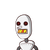# if x=3 is a root of quadratic equation Kx2 – kx-3=0, then find the value of k.​

if x=3 is a root of quadratic equation Kx2 – kx-3=0, then find the value of k.​

### 1 thought on “if x=3 is a root of quadratic equation Kx2 – kx-3=0, then find the value of k.​”

1.0,5

Step-by-step explanation:

given x=3

kx^2-kx-3=0

9k-3k-3=0

6k-3=0

k=1/2=0.5